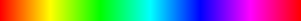## 3 novembre 2010

### Playing with HSV colors and HTML5

In a previous post I explained how to draw points on an HTML5 canvas. This time I will use this technique to draw colored bars.

The idea behind this post is to implement a color picker with HTML5 and Javascript.

The HSV color model

HSL and HSV are the two most common cylindrical-coordinate representations of points in an RGB color model, which rearrange the geometry of RGB in an attempt to be more perceptually relevant than the cartesian representation.

Citation from wikipedia
HSV stands for hue, saturation, and value.The hue can variate from 0 to 360°, the saturation and the value must be between 0 and 1. When the full range of these values is walked through, it generates a color cylinder as in the following image:

If a hue is selected (i.e. the main color is fixed), going through the range of S and L will produce a 2D slice of the cylinder.

Converting from HSV to RGB

Using the algorithm exposed in the above wikipedia page we can implement a function that will convert a color given in HSV to a RGB color.
```function colors.hsv_to_rgb(h, s, v) {
var c = v * s;
var h1 = h / 60;
var x = c * (1 - Math.abs((h1 % 2) - 1));
var m = v - c;
var rgb;

if (typeof h == 'undefined') rgb = [0, 0, 0];
else if (h1 < 1) rgb = [c, x, 0];
else if (h1 < 2) rgb = [x, c, 0];
else if (h1 < 3) rgb = [0, c, x];
else if (h1 < 4) rgb = [0, x, c];
else if (h1 < 5) rgb = [x, 0, c];
else if (h1 <= 6) rgb = [c, 0, x];

return [255 * (rgb + m), 255 * (rgb + m), 255 * (rgb + m)];
} ```

Selecting a color

The idea of the color picker is to show a bar with all the colors (hue).Once the color is choosed, we have to choose a saturation and value to refine the selection.Drawing a hue bar

Because we use the HSV color model it is very simple to draw the hue bar. We simply go through the possible values of the hue (0 to 360) using a saturation and a value of 1.
```// imgd represents the image data of the canvas object
function draw_horizontal_hue_bar(imgd, x, y, width, height) {
var inc = 360 / width;
for (var h = 0; h < 360; h += inc) {
for (var i = 0; i <= height; i++) {
var rgb = hsv_to_rgb(h, 1, 1);
point(imgd, x + Math.round(h/inc), y + i, rgb, rgb, rgb);
}
}
}

```

Drawing a slice of the color cylinder

Now that we have fixed hue let's draw a slice of the color cylinder in order to choose the saturation and the value. This is also very simple since we can go through the values of the saturation and value (0 to 1) keeping the hue fixed.
```function draw_sv_grid(imgd, hue, x, y, size) {
var inc = 1 / size;
for (var v = 0; v <= 1; v += inc) {
for (var s = 0; s <= 1; s += inc) {
var rgb = this.colors.hsv_to_rgb(hue, v, s);
this.point(imgd, x + Math.round(v * size), y + Math.round(s * size), rgb, rgb, rgb);
}
}
}

```
What's next
With the above algorithm we should have everything we need to implement an HSV color picker.

Some interesting problems remain though, as how to get the color of a pixel clicked in the canvas.

This will be for another post. Meanwhile, happy coding... ;-)

#### 1 commentaire:

1.These provided information was really so nice,thanks for giving that post and the more skills to develop after refer that post. Your articles really impressed for me,because of all information so nice.
school logo design uk